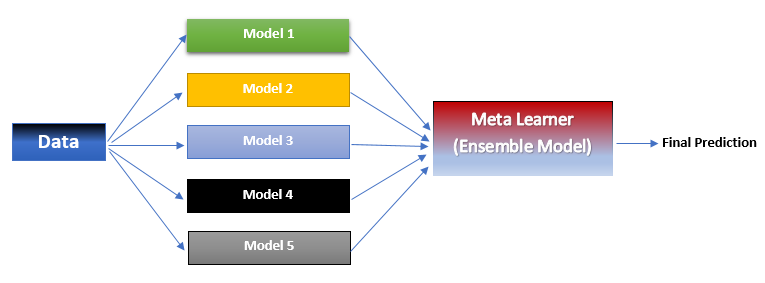....

Classification

Ensemble Technique

Machine Learning

Stacking

January 10, 2021

# Explain the working behind Stacking.

Stacking, also called as Stacked Generalization, stacks models on top of each other i.e. output of a model at lower level is used as input for the model at upper level. The lower level consists of two or more models(base models) and the out put from these models are fed to models at higher level(metal model).The purpose of stacking is to ensure that patterns in each subset of the training data have been properly learned. Let’s say that one of the base model constantly makes wrong predictions, then the meta model which receives output from it and other models(who do correct predictions) will also learn this and give less weightage to outputs of the aforementioned faulty model.

Let’s understand the steps involved in stacking using the following example: Say we have a simple stack generalization model with only two layer and the base layer constitutes of two models logistic regression and SVM classifier, with random Forest acting as a meta learner in the top layer. Following would be the steps involved in creation, training and validation of the aforementioned stacked generalization model|:

1. The dataset is split in the ratio 50:50. With x1, y1 and x2,y2 acting as the independent and dependent variables of the two subsets respectively.
2. Now we’ll further split the first subset into training and validation sets in the ration 80:20 respectively. Let’s call the independent and dependent variables of the training set as  as y1_train and x1_train respectively. Similarly,  the independent and dependent variables of the validation set will be referred as y1_test and x1_test respectively.
3. Now the logistic regression and SVM models will be trained on the training data i.e. x1_train and y1_train.
4. The models resulting from training in step 3 are validates using test set i.e. x1_test and y1_test. If their performance is satisfactory then we’ll proceed, else we’ll chose some other algorithm and repeat step 3.
5. The validated models would make prediction on dependent variables of second set i.e. x2. Since we have two base models we’ll get two set of predictions, say y’21 and y’22. Where y’21 and y’22 are prediction on x2 data by the logistic regression and SVM classifier algorithms respectively.
6. Now a new dataset would be created with y2 acting as dependent variable and y’21 & y’22 as  independent variables.
7. The meta learner model, her random Forest, will make predictions on the dataset created in step 6.
8. The resulting model from step 6 will be validated using validation data i.e. x1_test & y1_test

It is different from stacking in two aspects:

1. Each subset is trained using a different algorithm
2. Instead of aggregation a meta learner model is used for combining the outputs of different models.

by : Monis Khan

Quick Summary:

Stacking, also called as Stacked Generalization, stacks models on top of each other i.e. output of a model at lower level is used as input for the model at upper level. The lower level consists of two or more models(base models) and the out put from these models are fed to models at higher level(metal […]